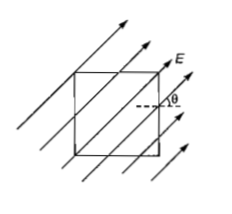NEET Physics Electric Charges and Fields Questions Solved

A surface of side L metre in the plane of the paper is placed in a uniform electric field E(volt/m) acting along the same place at an angle $\theta$ with the horizontal side of the square as shown in figure. The electric flux linked to the surface in unit of V-m, is(a) EL2                (b) EL2cos$\theta$

(c) EL2sin$\theta$          (d) 0

Concept Videos :-

#22 | Electric Flux
#23 | Gauss Theorem
#24 | Charged Infinite Wire (Gauss Theorem)
#25 | Charged Infinite Sheet (Gauss Theorem)
#26 | Charged Thin Conducting Shell (Gauss Theorem)
#27 | Solid Non Conducting Sphere : Gauss Theorem
#28 | Solid Sphere With Cavity : Gauss Theorem
#29 | Early Model of Atom (Gauss Theorem)
#30 | EFI Inside a Metal (Gauss Theorem)
#31 | Faraday Cages (Gauss Theorem)

Concept Questions :-

Gauss law
Explanation is a part of a Paid Course. To view Explanation Please buy the course.

Difficulty Level: